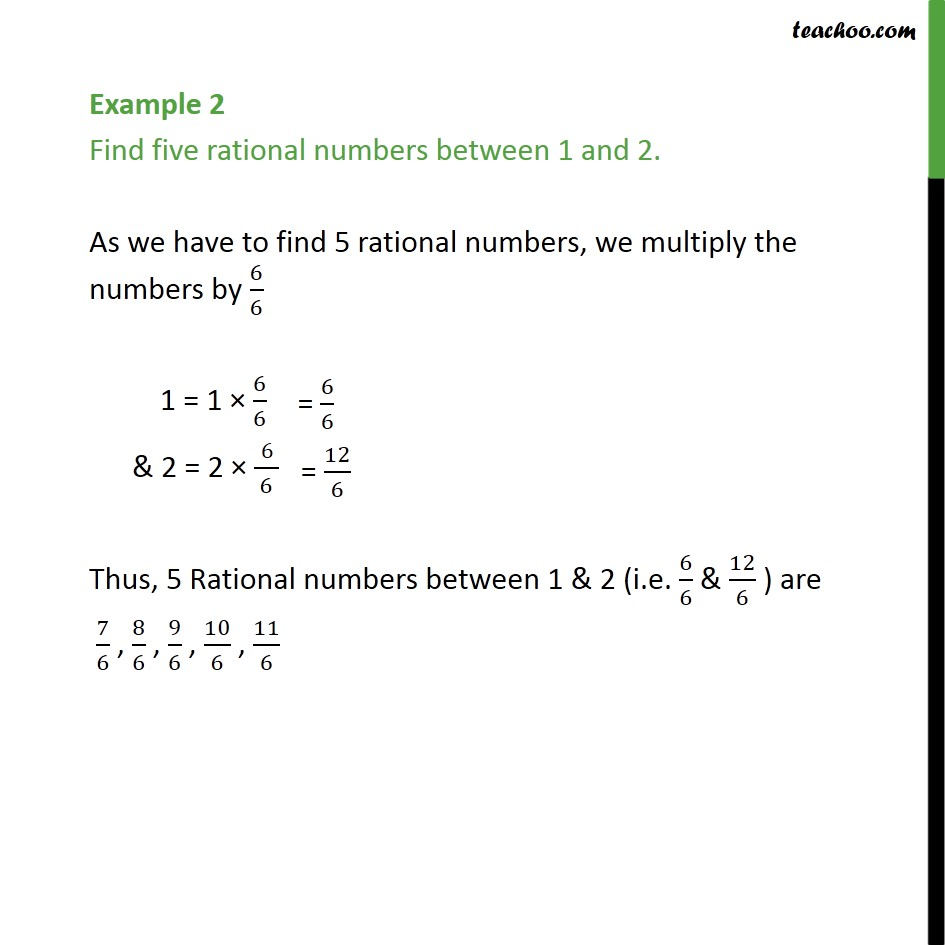Finding rational number between two numbers

Chapter 1 Class 9 Number Systems
Concept wiseLearn in your speed, with individual attention - Teachoo Maths 1-on-1 Class

### Transcript

Example 2 Find five rational numbers between 1 and 2. As we have to find 5 rational numbers, we multiply the numbers by 6/6 1 = 1 × 6/6 & 2 = 2 × (" " 6)/6 Thus, 5 Rational numbers between 1 & 2 (i.e. 6/6 & 12/6 ) are 7/6 , 8/6 , 9/6 , 10/6 , 11/6